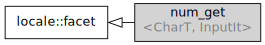/C++

# std::num_get

Defined in header `<locale>`
```template<
class CharT,
class InputIt = std::istreambuf_iterator<CharT>
> class num_get;```

Class `std::num_get` encapsulates the rules for parsing string representations of numeric values. Specifically, types `bool`, `unsigned short`, `unsigned int`, `long`, `unsigned long`, `long long`, `unsigned long long`, `float`, `double`, `long double`, and `void*` are supported. The standard formatting input operators (such as `cin >> n;`) use the `std::num_get` facet of the I/O stream's locale to parse the text representations of the numbers.Inheritance diagram.

### Type requirements

 -`InputIt` must meet the requirements of LegacyInputIterator.

### Specializations

Two standalone (locale-independent) full specializations and two partial specializations are provided by the standard library:

Defined in header `<locale>`
`std::num_get<char>` creates narrow string parsing of numbers
`std::num_get<wchar_t>` creates wide string parsing of numbers
`std::num_get<char, InputIt>` creates narrow string parsing of numbers using custom input iterator
`std::num_get<wchar_t, InputIt>` creates wide string parsing of numbers using custom input iterator

In addition, every locale object constructed in a C++ program implements its own (locale-specific) versions of these specializations.

### Member types

Member type Definition
`char_type` `CharT`
`iter_type` `InputIt`

### Member functions

 (constructor) constructs a new num_get facet (public member function) (destructor) destructs a num_get facet (protected member function) get invokes `do_get` (public member function)

### Member objects

 static std::locale::id id id of the locale (public member object)

### Protected member functions

 do_get [virtual] parses a number from an input stream (virtual protected member function)

### Example

```#include <iostream>
#include <locale>
#include <string>
#include <sstream>
#include <iterator>

int main()
{
std::string de_double = "1.234.567,89";
std::string us_double = "1,234,567.89";

// parse using streams
std::istringstream de_in(de_double);
de_in.imbue(std::locale("de_DE"));
double f1;
de_in >> f1;

std::istringstream us_in(de_double);
us_in.imbue(std::locale("en_US.UTF-8"));
double f2;
us_in >> f2;

std::cout << "Parsing " << de_double << " as double gives " << std::fixed
<< f1 << " in de_DE locale and " << f2 << " in en_US\n";

// use the facet directly
std::istringstream s3(us_double);
s3.imbue(std::locale("en_US.UTF-8"));
auto& f = std::use_facet<std::num_get<char>>(s3.getloc());
std::istreambuf_iterator<char> beg(s3), end;
double f3;
std::ios::iostate err;
f.get(beg, end, s3, err, f3);
std::cout << "parsing " << us_double
<< " as double using raw en_US facet gives " << f3 << '\n';
}```

Output:

```Parsing 1.234.567,89 as double gives 1234567.890000 in de_DE locale and 1.234000 in en_US
parsing 1,234,567.89 as double using raw en_US facet gives 1234567.890000```

 numpunct defines numeric punctuation rules (class template) num_put formats numeric values for output as character sequence (class template) operator>> extracts formatted data (public member function of `std::basic_istream`)